# Draw filled TEllipse objects with holes

Dear ROOTers,
I have used Root to analyse data for a few years already. Today I found a simple plotting problem for which I can’t find a solution online.
I’m trying to draw circles with “holes” on them. My aproach so far is trying to draw a TEllipse with a filling, but excluding parts of it.

The easiest way to put it is with an example. I’m trying to plot the following image (done with gimp) using ROOT: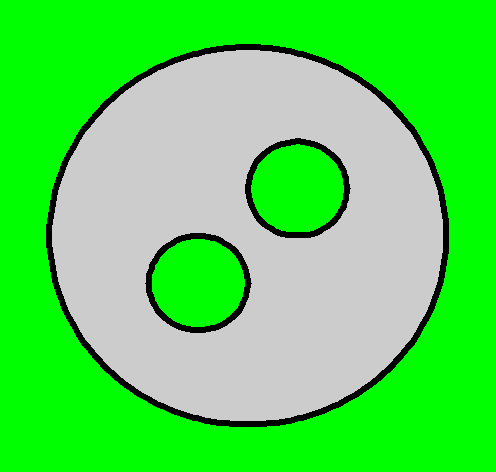The closest I get is this: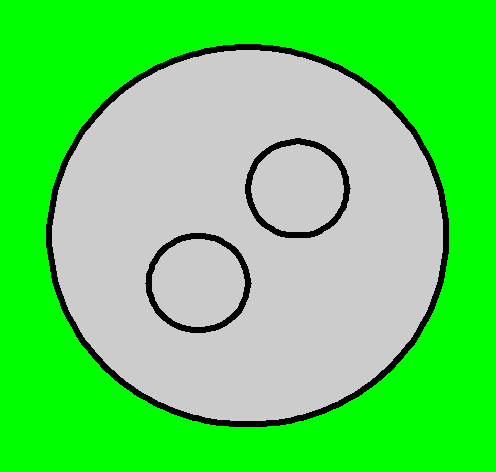Using this code:
circle_with_holes.C (627 Bytes)

As you can see, I plotted a green background and defined the large gray-filled circle and tried to plot hollow circles as the holes, but I still get the gray filling of the large circle, instead of the green of the background. Of course I didn’t expect this to work, but I thought it could be a good starting point for my problem.

I thought it could be possible to get the desired result (1st picture) if inverse TEllipse objects and TEllipse intersections and unions could be defined. If so, I could define the area to fill in gray as the intersection of the large circle with the union of the inverse of the 2 small circles. I already looked at the TEllipse and TAttFill documentation pages, but didn’t found any function that could help. I also had a look at the image gallery and found no similar images.
Any suggestions?
Thank you.

Regards,

mapaz

You can get the picture you want doing this:

``````{
TCanvas *c1 = new TCanvas("c1", "c1", 500, 500);
c1->Range(0, 0, 1, 1);

TEllipse *circle = new TEllipse(0.5, 0.5, 0.4);
circle->SetLineWidth(6);
//circle->SetFillStyle(1001); //solid
circle->SetFillColor(kGray);
circle->Draw("Lf");

TEllipse *hole1 = new TEllipse(0.4, 0.4, 0.1);
hole1->SetLineWidth(6);
hole1->SetFillColor(kGreen);
hole1->Draw("Lf");

TEllipse *hole2 = new TEllipse(0.6, 0.6, 0.1);
hole2->SetLineWidth(6);
hole2->SetFillColor(kGreen);
hole2->Draw("Lf");
}``````

Dear Couet,
thank you for your answer. Unfortunately your solution is not valid for my case, since filling the small circles is not what I wanted. The real case is not a simple bg, but a TH2 histogram. The region I want to shade is my ROI, which is defined as a circle with holes. So I really want to fill only the area of the inverse intersection of the union of the two small regions.

Here I’m posting a more realistic example:

Plot: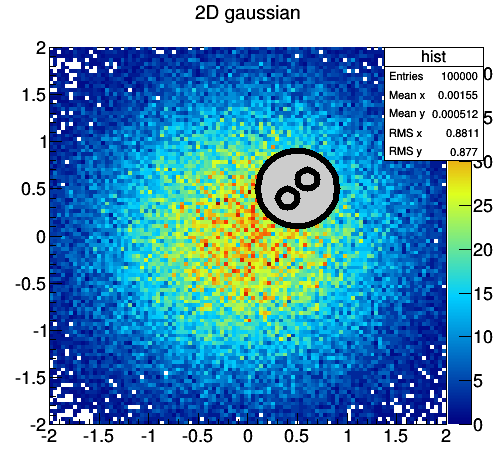Code:
circle_with_holes.C (1.17 KB)

Any suggestions?
Thank you.
Regards,

mapaz

I see … yes it is different … No idea yet …

Would it help if I added a function that takes (x, y) coordinates and determines if they are inside my ROI or not? The problem is reduced to pass this information to TAttFill then, which I don’t know how to do.
I also don’t mind defining the circles as TGraphs or TPolyLines or smthg else if it helps with the drawing.

Seems to me the only way is to draw the filled area as one single polygon (or may be 2)

Thanks for the suggestion. I found an acceptable workaround for my case: draw first the circles (filling the bg color for the holes, as you suggested in the 1st post), then plot the histogram on top using only contour lines.

Hi, you can do it by setting FillStyle to 0
for instance:

``````{
TEllipse *ellipse = new TEllipse(0.5,0.5,0.1,0.1);
ellipse->SetFillStyle(0);
ellipse->SetLineWidth(3);
}
``````

maybe is too late but anyway.

Cheers

Dear Orlando,
thank you for your interest in the topic. The FillStyle 0 for the ellipse marking the hole does not solve the problem (I’m already using it in my examples), since you still see the background of the larger ellipse, instead of the underlying distribution.
Cheers

Dear ROOTers,
in order to be specific, this is a simple example of what I want to achieve (done using GIMP):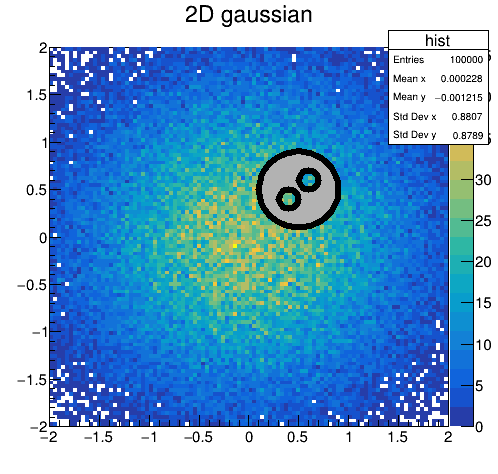But I can’t find an easy way of doing it with ROOT. I can either have all circles in gray: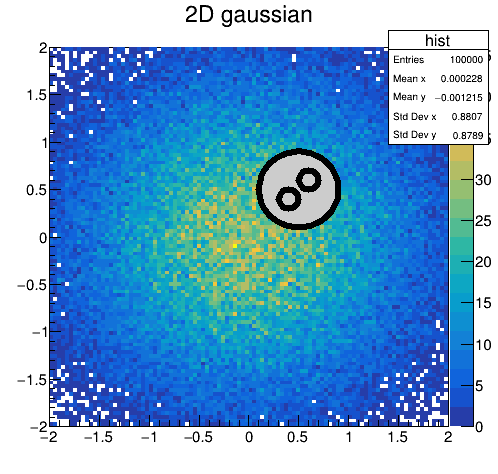using this code: circle_with_holes.C (1.2 KB)

or all circles transparent (no fill):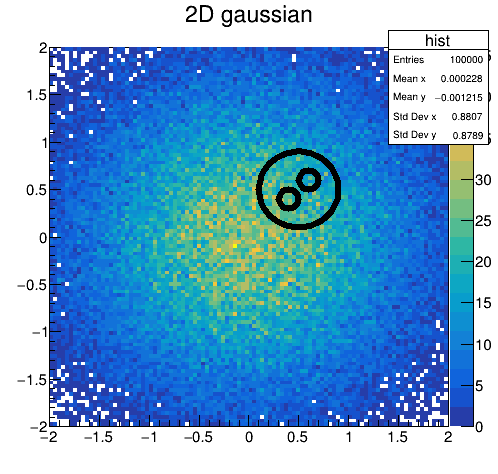using this code: circle_with_holes_transparent.C (1.2 KB)

Regards,

mapaz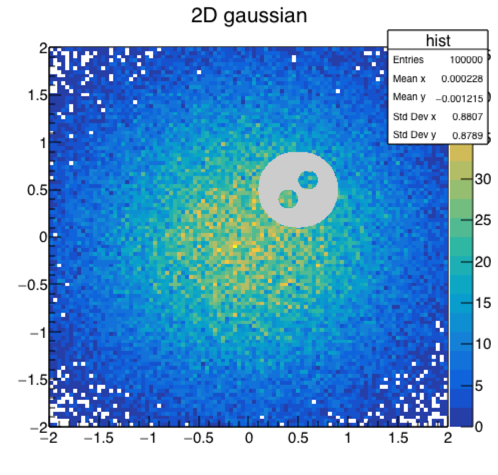``````//2D gaussian function
Double_t Gaus2D(Double_t *x, Double_t *par) {
Double_t r1 = Double_t((x-par)/par);
Double_t r2 = Double_t((x-par)/par);
return par*TMath::Exp(-0.5*(r1*r1+(par*r1*r2)+r2*r2));
}

void AddCircularArcToTCutG(TCutG* cut, double x, double y, double r, double angStart=0, double angStop=360) {
const int numPoints = 100;
double angCovered = angStop - angStart;
for (int i=0;i<numPoints;i++) {
double angle = angStart + (double) i / (numPoints-1) * angCovered;
cut->SetPoint(cut->GetN(), r * cos(angle) + x, r * sin(angle) + y);
}
}

//Draw circle with holes
TCanvas *circle_with_holes(){
TCanvas *c1 = new TCanvas("c1", "c1", 500, 500);
c1->cd();

//define 2D gaussian function
TF2* fgaus2d = new TF2("gaussian_fill", &Gaus2D, -3, 3, -3, 3, 6);
fgaus2d->SetParameters(1.,0,1,0,1,0);
fgaus2d->SetParNames("norm","xmean","xsigma","ymean","ysigma","orientation");

//fill random gaussian histogram
TH2D* hist = new TH2D("hist","2D gaussian",100,-2,2,100,-2,2);
hist->FillRandom("gaussian_fill", 100000);
hist->Draw("colz");

TCutG *cut = new TCutG("Shape");
//First hole has to be drawn opposite (CW) to others (CCW) to ensure that the
//  line does not cross itself.
AddCircularArcToTCutG(cut, 0.5, 0.5, 0.4, 0, -360);
AddCircularArcToTCutG(cut, 0.6, 0.6, 0.1, -90, 270);
cut->SetPoint(cut->GetN(), cut->GetX(), cut->GetY());
cut->SetFillColor(kGray);
cut->Draw("F SAME");

return c1;
}

``````

There are a number of different way the arcs can be added together. Some of them will lead to lines that cross each other and the fill would produce unexpected results. For example this would work as well:

``````  AddCircularArcToTCutG(cut, 0.5, 0.5, 0.4, 0, -360);
AddCircularArcToTCutG(cut, 0.6, 0.6, 0.1, 0, 180);